Latest Banking jobs   »

# Reasoning Ability Quiz For SIDBI GRADE A 2023- 12th January

Direction (1-3): Study the information carefully and answer the questions accordingly.
Point A is 6m west of point B. Point B is 10m north of point C. Point D is 5m south of point E and 12m west of point C. Point E is 6m west of point F. Point F is 10m north of point G.

Q1. Point F is in which direction of point B?
(a) South
(b) North
(c) South – east
(d) South – west
(e) None of these

Q2. What is the shortest distance between the points A and F?
(a) 7m
(b) 6m
(c) 5m
(d) 4m
(e) None of these

Q3. Point E is in which direction of point C?
(a) North
(b) South
(c) North-west
(d) North-east
(e) None of these

Direction (4-6): Study the information carefully and answer the questions accordingly.
There are nine members in a family with two married couples and three generations. B is the grandfather of H who is the nephew of I. K is the daughter of E. D is the brother-in-law of F who is unmarried. G is the father of E. There are four females. C is the mother of I who is the sister-in-law of E. I is unmarried. B has only one daughter.

Q4. How is C related to K?
(a) Grandmother
(b) Sister
(c) Aunt
(d) Mother
(e) None of these

Q5. How is I related to H?
(a) Uncle
(b) Mother
(c) Aunt
(d) Sister
(e) None of these

Q6. Find the correct statement from the following?
(a) G is the father of I
(b) H and K are not siblings
(c) E is the sister-in-law of F
(d) B is the father of F
(e) E is mother of H

Direction (7-8): Study the following information carefully and answer the questions accordingly.
Point P is 20m north of point N. Point Y is 20m south of point Z and 15m east of point W. Point Q is 10m west of point N. Point W is 15m south of point Q.

Q7. Point Q is in which direction of point Z?
(a) South
(b) North-East
(c) South-West
(d) West
(e) None of these

Q8. What is the shortest distance between points Z and N?
(a) 12m
(b) 5√7m
(c) 5√2m
(d) 9m
(e) None of these

Directions (9-11): Study the following information carefully and answer the given questions.
Amongst five persons, P, R, S, T and U, each has different weight. P’s weight is more than R and T. S weight is less than U. Only two persons weight is more than P whose weight is 65kg. Youngest person weight is 55kg.

Q9. Who among the following is youngest person?
(a) U
(b) R
(c) S
(d) T
(e) Can’t be determined

Q10. If U’s weight is 70kg, then what may be the weight of S?
(a) 63
(b) 64
(c) 68
(d) 72
(e) None of these

Q11. How many persons are heavier than R?
(a) One
(b) Two
(c) Three
(d) None
(e) Can’t be determined

Direction (12-13): Read the following information carefully and answer the questions given below.
There are eight members i.e., P, Q, R, S, T, U, V, and W in a family of three generations and there are three married couples. R is an unmarried person. Q is the mother-in-law of S. U is the brother-in-law of W who has no sibling. T is the aunt of R who is the daughter of U. U is the child of P who has one granddaughter and one grandson. U has only one sister and no brother. W is the father of V.

Q12. What is the relation of V with respect to P?
(a) Son
(b) Son-in-law
(c) Grandson
(d) Brother-in-law
(e) None of these

Q13. Who amongst the following person is the sister-in-law of T?
(a) Q
(b) S
(c) R
(d) Cannot be determined
(e) None of these

Direction (14-15): Study the information carefully and answer the questions accordingly.
Rani starts walking towards the south to reach point W after a distance of 30m. Now, she took a left turn and reached point H after a distance of 20m. From here, she took a left turn and covered a distance of 15m to reach point R. Finally, she turned towards the east and covered a distance of 35m to reach point Z.

Q14. What is the shortest distance between the points W and Z?
(a) 65m
(b) 5√110m
(c) 40m
(d) 5√130m
(e) None of these

Q15.Point H is in which direction of Rani’s starting point?
(a) South
(b) West
(c) South-East
(d) North-West
(e) None of these

Solutions

Solution (1-3):
Sol.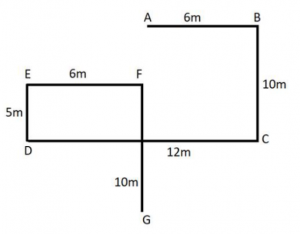S1 Ans. (d)
S2 Ans. (c)
S3 Ans. (c)

Solution (4-6):
Sol.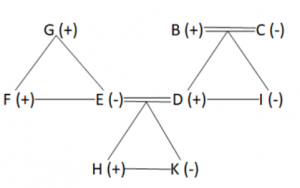S4 Ans. (a)
S5 Ans. (c)
S6 Ans. (e)

Solution (7-8):
Sol.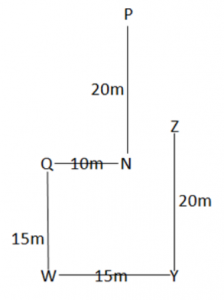S7 Ans. (c)
S8 Ans. (c)

Solutions (9-11):
Sol. The arrangement will be: U>S>P>R/T>T/R

S9. Ans. (e)
S10. Ans. (c)
S11. Ans. (e)

Solution (12-13):
Sol.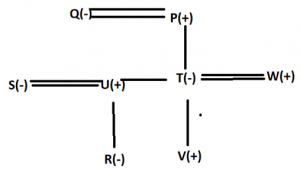S12. Ans. (c)
S13. Ans. (b)

Solution (14-15):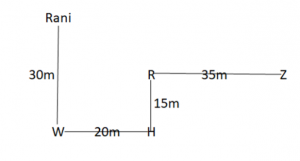S14. Ans. (d)
S15. Ans. (c)## FAQs

### When will the SIDBI Grade A Exam 2023 be held?

The SIDBI Grade A Exam 2023 will be held on 28th January 2023.

#### Congratulations!